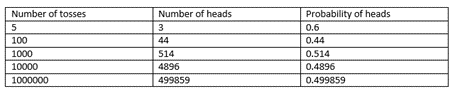Access Point #: MAFS.912.S-IC.1.AP.2b

Approximate the probability of a chance event by collecting data on the chance process that produces it and observing its long-run relative frequency, and predict the approximate relative frequency given the probability. For example, when rolling a number cube 600 times, predict that a 3 or 6 would be rolled roughly 200 times, but probably not exactly 200 times.
Clarifications:

Essential Understandings

Concrete:

• Use items like coins to create events.
• Using manipulatives and a chart to capture the outcomes of coin flips or dice rolls.
• Use items like coins to determine the probability of an outcome (1/2 heads).
Representation:
• Understand the following concepts, symbols, and vocabulary for: probability and likelihood.
• Identify the formula for finding probability of an event (probability of an event happening = number of ways it can happen/total number of outcomes).General Information
Number: MAFS.912.S-IC.1.AP.2b
Category: Access Points
Cluster: Understand and evaluate random processes underlying statistical experiments. (Algebra 2 - Supporting Cluster)

Clusters should not be sorted from Major to Supporting and then taught in that order. To do so would strip the coherence of the mathematical ideas and miss the opportunity to enhance the major work of the grade with the supporting clusters.

Related Standards

This access point is an alternate version of the following benchmark(s).

Related Courses

This access point is part of these courses.
1200330: Algebra 2
1200340: Algebra 2 Honors
1207310: Liberal Arts Mathematics
1210300: Probability and Statistics Honors
2000520: Bioscience 3 Honors
1200335: Algebra 2 for Credit Recovery
2100365: African History Honors
7912095: Access Algebra 2

Related Resources

Vetted resources educators can use to teach the concepts and skills in this access point.

Student Resources

Vetted resources students can use to learn the concepts and skills in this access point.

Parent Resources

Vetted resources caregivers can use to help students learn the concepts and skills in this access point.